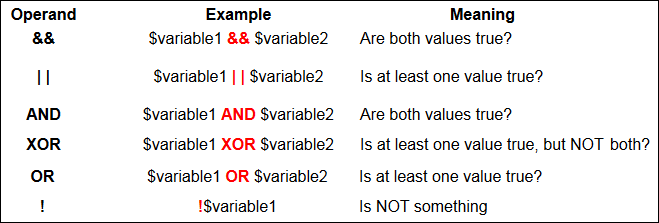Home and Learn: PHP Programming Course

# PHP Logical Operators

As well as the PHP comparison operators you saw earlier, there's also something called Logical Operators. You typically use these when you want to test more than one condition at a time. For example, you could check to see whether the username and password are correct from the same If Statement. Here's the table of these Operands.The new Operands are rather strange, if you're meeting them for the first time. A couple of them even do the same thing! They are very useful, though, so here's a closer look.

### The && Operator

The && symbols mean AND. Use this if you need both values to be true, as in our username and password test. After all, you don't want to let people in if they just get the username right but not the password! Here's an example:

print("Welcome back!");

}
else {

}

The if statement is set up the same, but notice that now two conditions are being tested:

This says, "If username is correct AND the password is ok, too, then let them in". Both conditions need to go between the round brackets of your if statement.

### The | | Operator

The two straight lines mean OR. Use this symbol when you only need one of your conditions to be true. For example, suppose you want to grant a discount to people if they have spent more than 100 pounds OR they have a special key. Else they don't get any discount. You'd then code like this:

\$total_spent =100;
\$special_key ='SK12345';

if (\$total_spent ==100 | | \$special_key =='SK12345') {

print("Discount Granted!");

}
else {

print("No discount for you!");

}

This time we're testing two conditions and only need ONE of them to be true. If either one of them is true, then the code gets executed. If they are both false, then PHP will move on.

### AND and OR

These are the same as the first two! AND is the same as && and OR is the same as ||. There is a subtle difference, but as a beginner, you can simply replace this:

With this

And this:

\$total_spent ==100 | | \$special_key =='SK12345'

With this:

\$total_spent ==100 OR \$special_key =='SK12345'

It's up to you which you use. AND is a lot easier to read than &&. OR is a lot easier to read than ||.

The difference, incidentally, is to do with Operator Precedence. We touched on this when we discussed variables, earlier. Logical Operators have a pecking order, as well. The full table is coming soon!

### XOR

You probably won't need this one too much. But it's used when you want to test if one value of two is true but NOT both. If both values are the same, then PHP sees the expression as false. If they are both different, then the value is true. Suppose you had to pick a winner between two contestants. Only one of them can win. It's an XOR situation!

\$contestant_one = true;
\$contestant_two = true;

if (\$contestant_one XOR \$contestant_two) {

print("Only one winner!");

}
else {

print("Both can't win!");

}

See if you can guess which of the two will print out, before running the script.

The ! Operator
This is known as the NOT operator. You use it test whether something is NOT something else. You can also use it to reverse the value of a true or false value. For example, you want to reset a variable to true, if it's been set to false, and vice versa. Here's some code to try:

\$test_value = false;

if (\$test_value == false) {

print(!\$test_value);

}

The code above will print out the number 1! (You'll see why when we tackle Boolean values below.) What we're saying here is, "If \$test_value is false then set it to what it's NOT." What it's NOT is true, so it will now get this value. A bit confused? It's a tricky one, but it can come in handy!

In the next part, we'll take a look at Boolean values.

Back to the PHP Contents Page

Email us: enquiry at homeandlearn.co.uk